output.to from Sideway
Number Theory

Factorization

Draft for Information Only

# Content

``` Factorization   Prime Factorization Trial Division   Fermat Factorization   Pollard Rho Factorization ```

# Factorization

Factorization is one of the methods to verify whether a number is prime.

## Prime Factorization Trial Division

The direct search factorization is a bruce force factorization method for determining all possible prime factors of a composite number, N, through the repeated examination of divisibility by  trial division from a set of non-trivial prime divisors.

Let x, y are non-trivial factors of integer N with N=xy. imply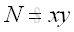It is not necessary to test all numbers from 1 to N-1.

If x, y are non-trivial factors of integer N with N=xy and x≤y, then x≤√N.

Since if x>√N, then y≥x>√N and imply xy>N, which contradicts to the assumption N=xy.

Therefore, the trial division can be performed by checking whether x divides N, x|N, for x=2 to the floor of √N. if x is found, imply x≡0 (mod N),  and y, y=N/x is a factor also.

In verifying whether a number N is prime,  x can be limited to a non-trivial prime factor.

## Fermat Factorization

The Fermat factorization (1600s) from Pierre de Fermat is another way of factoring a composite number by considering the composite number as the difference of squares.  This standard binomial can then be factorized into a product. Imply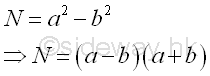Since all even number can be divided by 2, let x, y are non-trivial odd factors of integer N with N=xy. implyEquate two equations. Imply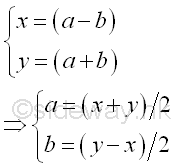Both a and b are integers because x and y are odd integers, the sum and difference between any two odd number are even number which is divisible by 2. As x and y are non trivial factors,  √N≥x>1 and y≥x, imply a≥1 and b≥0.

Instead of testing the non trivial factors x, and y, Fermat factorization examine the integers a and b. Imply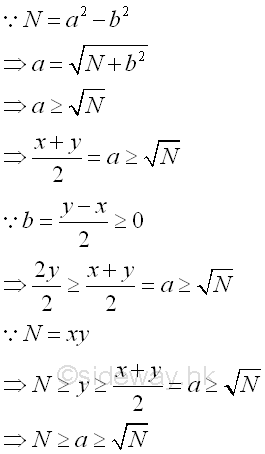Therefore, the Fermat factorization can be performed by checking a from the floor of √N to N. whether the corresponding value of b is an integer and the corresponding terms of the product, (a-b) and (a+y) are the non trivial factors of N. Imply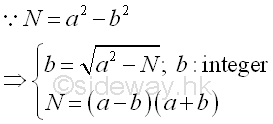In verifying whether a number N is prime,  the value of integer a should be choosen from the floor of √N to N.

## Pollard Rho Factorization

The Pollard rho factorization or Pollard's Monte Carlo factorization method (1975) from J.M. Pollard is another technique of finding factors of a composite number by making use of probabilistic ideas from transforming a sequencial sequence to a congruential pseudo-random sequence to increase the probability of getting prime factors of the composite number.

©sidewayReferences

1. R. Paulo, 1996, The New Book of Prime Number Records, Springer-Verlag, New York
2. Wolstenholme, R.J., 1862, On Certain Properties of Prime Numbers, The Quarterly Journal of Pure and Applied Mathematics, Vol 5, p35-39, London
3. Mann, H.B., Shanks D., 1972, A Necessary and Sufficient Condition for Primality, and Its Source, Journal of Combinatorial Theory, 13, p131-134
4. J.M Pollard, Kangaroos, 1975, A Monte Carlo Method for Factorization, BIT 15 (1975), 331-334ID: 120400011 Last Updated: 2012/4/17 Revision: Ref:Home (5)

Business

Management

HBR (3)

Information

Recreation

Hobbies (7)

Culture

Chinese (1097)

English (336)

Reference (66)

Computer

Hardware (149)

Software

Application (187)

Digitization (24)

Numeric (19)

Programming

Web (648)CSS (SC)

ASP.NET (SC)

HTML

Knowledge Base

Common Color (SC)

Html 401 Special (SC)

OS (389)

MS Windows

Windows10 (SC)

.NET Framework (SC)

DeskTop (7)

Knowledge

Mathematics

Formulas (8)

Number Theory (206)

Algebra (20)

Trigonometry (18)

Geometry (18)

Calculus (67)

Complex Analysis (21)

Engineering

Tables (8)

Mechanical

Mechanics (1)

Rigid Bodies

Statics (92)

Dynamics (37)

Fluid (5)

Control

Acoustics (19)

Biology (1)

Geography (1)

Copyright © 2000-2019 Sideway . All rights reserved Disclaimers last modified on 10 Feb 2019